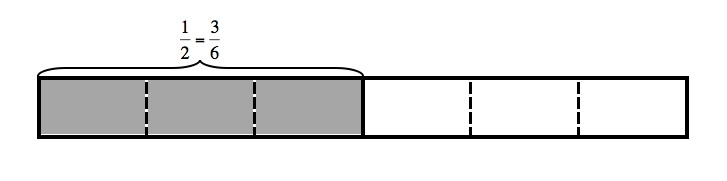Jon and Charlie's Run

Alignments to Content Standards: 3.NF.A.3.a

Jon and Charlie plan to run together. They are arguing about how far to run. Charlie says,

I run $\frac{3}{6}$ of a mile each day.

Jon says,

I can only run $\frac{1}{2}$ of a mile.

If Charlie runs $\frac{3}{6}$ of a mile and Jon runs $\frac12$ of a mile, explain why it is silly for them to argue. Draw a picture or a number line to support your reasoning.

IM Commentary

The purpose of this task is to present students with a context where they need to explain why two simple fractions are equivalent and is most appropriate for instruction. Students can illustrate the fact that the two fractions are equivalent by showing they belong at the same point on the number line or are represented by the same area in an appropriate diagram. Students will benefit from discussing their reasoning in small groups or as a class.

Solution

It is silly for Jon and Charlie to argue about the length of their run because the two lengths that each boy will run are equal. Consider the rectangle below that has a length of 1 which represents 1 mile. It is divided into 6 equal pieces and 3 are shaded. So the 3 shaded pieces represent $\frac36$ of a mile.Since all 6 pieces are the same length, the three shaded pieces together have the same length as the three unshaded pieces, so we know that the shaded area also represents $\frac12$ mile. We can also see this equivalence when we plot $\frac12$ and $\frac36$ on a number line. If we place 5 tick marks at even intervals between 0 and 1, we will divide the segment between 0 and 1 into 6 equal length pieces. $\frac36$ will be plotted at the third tick mark to the right of 0.This tick mark also marks the half-way point of the segment between 0 and 1, so we will also plot $\frac12$ there. This means that these two fractions are equivalent.

So Jon and Charlie will both run the same distance and can run together the whole way.